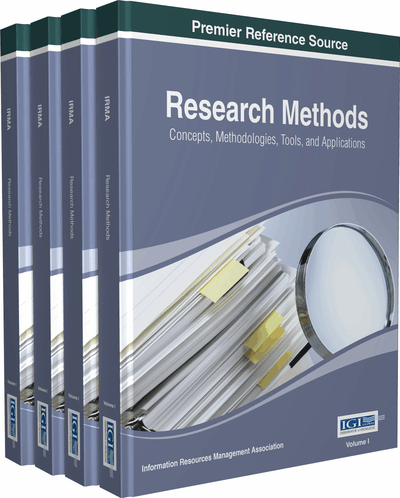# Interval Type-Two Fuzzy Logic for Quantitatively Defining Imprecise Linguistic Terms in Politics and Public Policy

Ashu M. G. Solo (Maverick Technologies America Inc., USA)
Copyright: © 2015 |Pages: 9
DOI: 10.4018/978-1-4666-7456-1.ch030

## Abstract

The previous chapter describes the use of type-one fuzzy logic for quantitatively defining imprecise linguistic terms in politics and public policy. This chapter shows the use of interval type-two fuzzy logic for this purpose and the advantages and disadvantages of using interval type-two fuzzy logic instead of type-one fuzzy logic for this purpose. Interval type-two fuzzy sets can be used to properly ask and answer queries about quantitatively defining imprecise linguistic terms in politics and public policy like “rich,” “middle class,” “poor,” “low inflation,” “medium inflation,” and “high inflation.” Imprecise terms like these in natural languages should be considered to have “qualitative definitions,” “quantitative definitions,” “crisp quantitative definitions,” “fuzzy quantitative definitions,” “type-one fuzzy quantitative definitions,” and “interval type-two fuzzy quantitative definitions.”
Chapter Preview
Top

## 1. Introduction

The previous chapter (Solo, 2014a) describes the use of type-one fuzzy logic for quantitatively defining imprecise linguistic terms in politics and public policy. This chapter describes the use of interval type-two fuzzy logic for the same purpose. Also, this chapter shows the advantages and disadvantages of using interval type-two fuzzy logic instead of type-one fuzzy logic for quantitatively defining imprecise linguistic terms in politics and public policy. This chapter assumes that one has read the previous chapter (Solo, 2014a).

Type-two fuzzy logic can be used to properly quantitatively define many imprecise linguistic terms used in politics and public policy including income levels, unemployment levels, inflation, voter turnout levels, etc. Type-two fuzzy logic can be used to quantitatively define imprecise linguistic terms used in politics like poor, middle class, very poor, extremely poor, high inflation, medium inflation, low inflation, high unemployment, moderate unemployment, low unemployment, extremely high unemployment, extremely low inflation, high voter turnout, moderate voter turnout, low voter turnout, etc. Interval type-two fuzzy sets in fuzzy logic have been used for imprecise linguistic terms in many intelligent systems applications, but this research paper proposes the use of interval type-two fuzzy sets for the application of asking and answering queries about quantitatively defining imprecise linguistic terms in natural languages. This research paper describes how interval type-two fuzzy sets can specifically be used in asking and answering queries about defining the imprecise linguistic terms rich, middle class, and poor.

The use of fuzzy logic in quantitatively defining imprecise linguistic terms in politics is an example of political engineering and computational politics. These fields were defined by Ashu M. G. Solo in a chapter of this book (Solo, 2014b) and an earlier research paper (Solo, 2011). The use of fuzzy logic in quantitatively defining imprecise linguistic terms in public policy is an example of public policy engineering and computational public policy. These fields were defined by Solo in another chapter of this book (Solo, 2014c) and an earlier research paper (Solo, 2011).

A type-two fuzzy set allows the inclusion of uncertainty into the parameters of a membership function. The membership function of a type-two fuzzy set is in itself a fuzzy set. A type-two fuzzy set is three-dimensional where the third dimension indicates the degree of membership of the two-dimensional membership function at each point in its two-dimensional domain.

In a type-two fuzzy set, a footprint of uncertainty indicates the upper and lower bounds in the two-dimensional domain of a type-two fuzzy set. A footprint of uncertainty in a type-two fuzzy set is a region bounded by an upper membership function and lower membership function.

An interval type-two fuzzy set is a type-two fuzzy set in which the third dimension is constant in value meaning the degree of membership is constant for the two-dimensional membership function at each point in its two-dimensional domain. Therefore, the third dimension is ignored.

It would be extremely difficult to linguistically describe an imprecise linguistic term with a type-two fuzzy set because there is a third dimension that indicates the degree of membership of the two-dimensional membership function at each point in its two-dimensional domain. It is much less difficult to linguistically describe an imprecise linguistic term with an interval type-two fuzzy set because the third dimension is constant in value and can be ignored. Because it is impractical to attempt to linguistically describe a type-two fuzzy set for an imprecise linguistic term, this research chapter only covers the usage of interval type-two fuzzy sets for describing imprecise linguistic terms.

## Complete Chapter List

Search this Book:
Reset